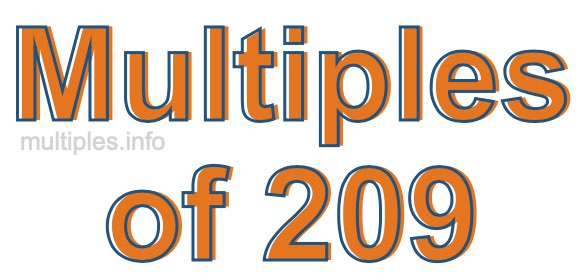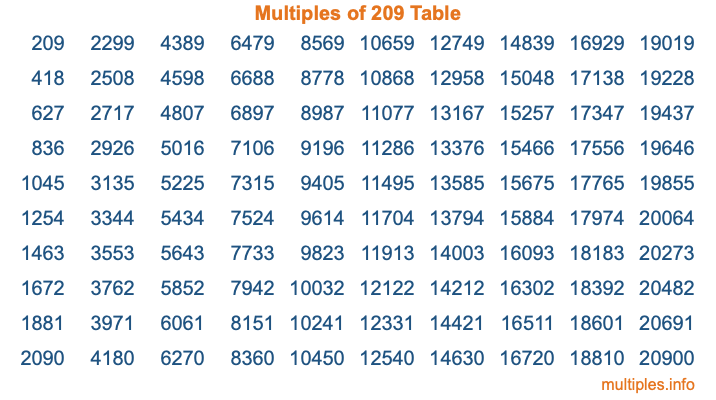Multiples of 209Welcome to the Multiples of 209 page. Here we will first teach you everything you will ever need to know about the multiples of 209, and then give you a study guide summary of everything we taught you to make sure you remember it all. Use this page to look up facts and learn information about the multiples of 209. This page will make you a multiples of two hundred nine expert!

Definition of Multiples of 209
Multiples of 209 are all the numbers that when divided by 209 equal an integer. Each of the multiples of 209 are called a multiple. A multiple of 209 is created by multiplying 209 by an integer.

Therefore, to create a list of multiples of 209, you start with 1 multiplied by 209, then 2 multiplied by 209, then 3 multiplied by 209, and so on for as long as you want. Thus, the list of the first five multiples of 209 is 209, 418, 627, 836, and 1045. To see a larger list of multiples of 209, see the printable image of Multiples of 209 further down on this page. We also have a category where you can choose any nth multiple of 209.

Multiples of 209 Checker
The Multiples of 209 Checker below checks to see if any number of your choice is a multiple of 209. In other words, it checks to see if there is any number (integer) that when multiplied by 209 will equal your number. To do that, we divide your number by 209. If the the quotient is an integer, then your number is a multiple of 209.

Is  a multiple of 209?

Least Common Multiple of 209 and ...
A Least Common Multiple (LCM) is the lowest multiple that two or more numbers have in common. This is also called the smallest common multiple or lowest common multiple and is useful to know when you are adding our subtracting fractions. Enter one or more numbers below (209 is already entered) to find the LCM.

Check out our LCM Calculator if you need more details about the Least Common Multiple or if you need the LCM for different numbers for adding and subtraction fractions.

nth Multiple of 209
As we stated above, 209 is the first multiple of 209, 418 is the second multiple of 209, 627 is the third multiple of 209, and so on. Enter a number below to find the nth multiple of 209.

th multiple of 209

Multiples of 209 vs Factors of 209
209 is a multiple of 209 and a factor of 209, but that is where the similarities end. All postive multiples of 209 are 209 or greater than 209. All positive factors of 209 are 209 or less than 209.

Below is the beginning list of multiples of 209 and the factors of 209 so you can compare:

Multiples of 209: 209, 418, 627, 836, 1045, etc.

Factors of 209: 1, 11, 19, 209

As you can see, the multiples of 209 are all the numbers that you can divide by 209 to get a whole number. The factors of 209, on the other hand, are all the whole numbers that you can multiply by another whole number to get 209.

It's also interesting to note that if a number (x) is a factor of 209, then 209 will also be a multiple of that number (x).

Multiples of 209 vs Divisors of 209
The divisors of 209 are all the integers that 209 can be divided by evenly. Below is a list of the divisors of 209.

Divisors of 209: 1, 11, 19, 209

The interesting thing to note here is that if you take any multiple of 209 and divide it by a divisor of 209, you will see that the quotient is an integer.

Multiples of 209 Table
Below is an image of the first 100 multiples of 209 in a table. The table is in chronological order, column by column. The first column has the first ten multiples of 209, the second column has the next ten multiples of 209, and so on.The Multiples of 209 Table is also referred to as the 209 Times Table or Times Table of 209. You are welcome to print out our table for your studies.

Negative Multiples of 209
Although not often discussed or needed in math, it is worth mentioning that you can make a list of negative multiples of 209 by multiplying 209 by -1, then by -2, then by -3, and so on, to get the following list of negative multiples of 209:

-209, -418, -627, -836, -1045, etc.

Multiples of 209 Summary
Below is a summary of important Multiples of 209 facts that we have discussed on this page. To retain the knowledge on this page, we recommend that you read through the summary and explain to yourself or a study partner why they hold true.

There are an infinite number of multiples of 209.

A multiple of 209 divided by 209 will equal a whole number.

209 divided by a factor of 209 equals a divisor of 209.

The nth multiple of 209 is n times 209.

The largest factor of 209 is equal to the first positive multiple of 209.

209 is a multiple of every factor of 209.

209 is a multiple of 209.

A multiple of 209 divided by a divisor of 209 equals an integer.

209 divided by a divisor of 209 equals a factor of 209.

Any integer times 209 will equal a multiple of 209.

Multiples of a Number
Here you can get the multiples of another number, all with the same attention to detail as we did for multiples of 209 on this page.

Multiples of
Multiples of 210
Did you find our page about multiples of two hundred nine educational? Do you want more knowledge? Check out the multiples of the next number on our list!

Copyright  |   Privacy Policy  |   Disclaimer  |   Contact